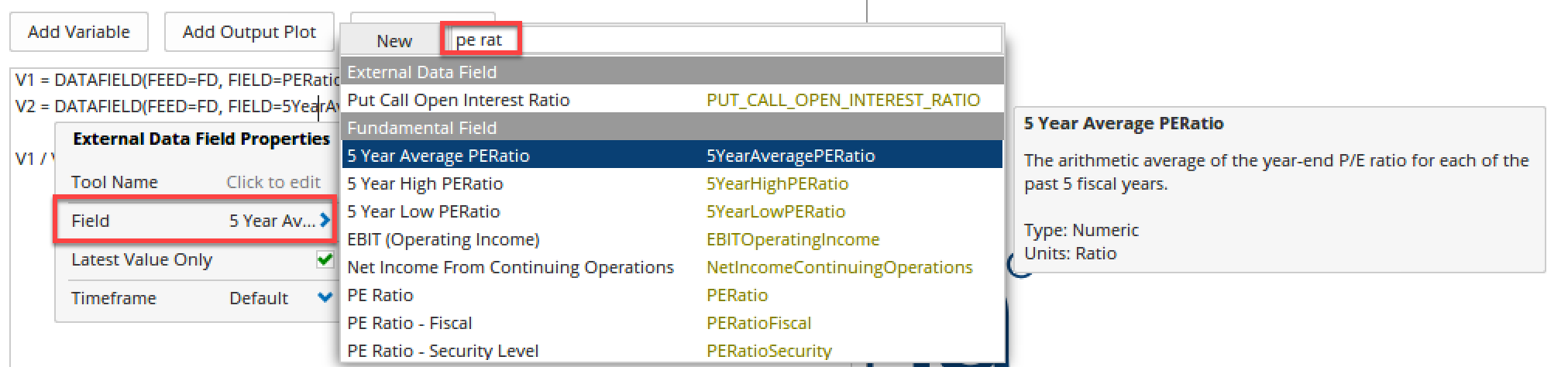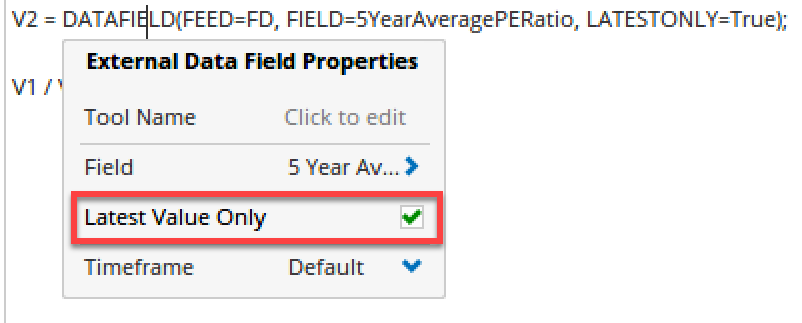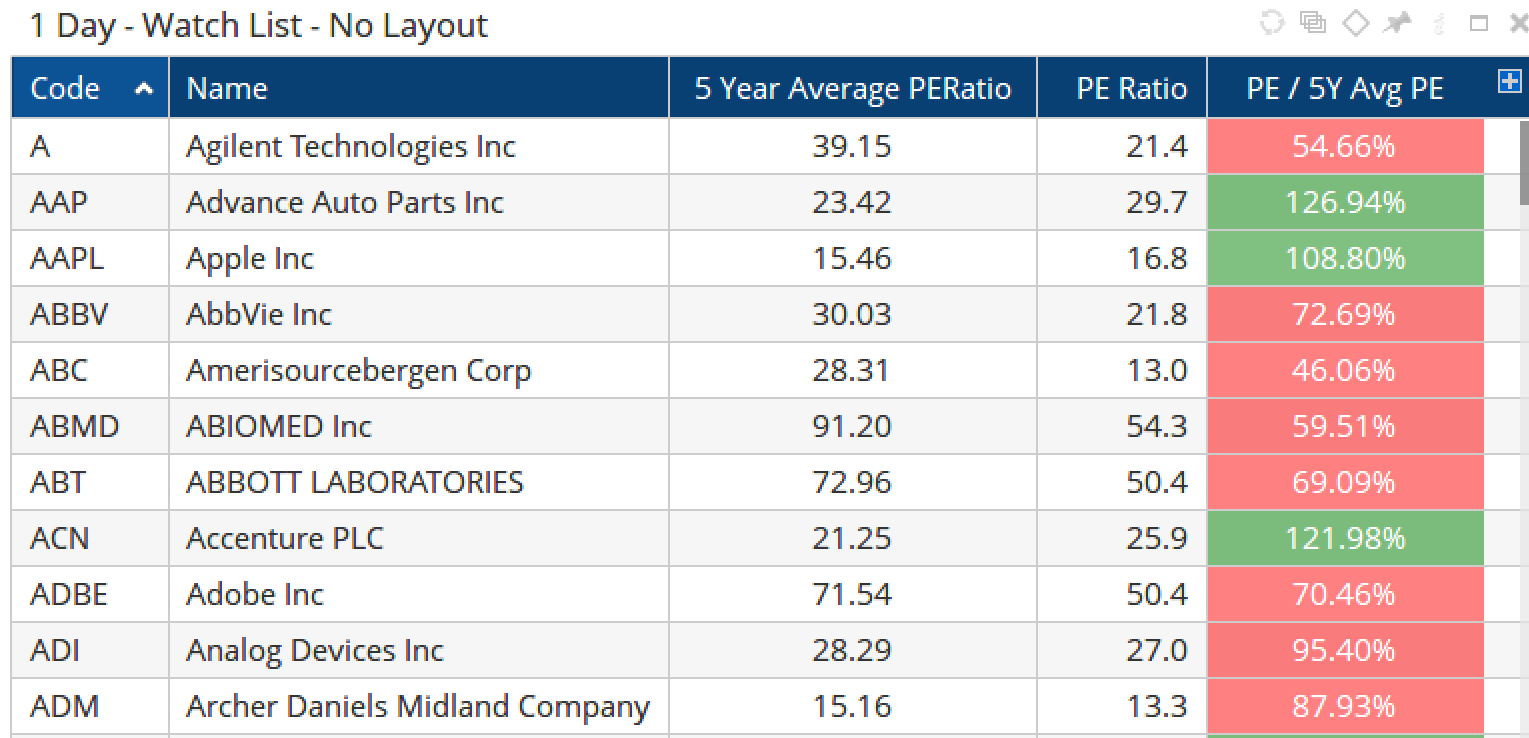# DataField() Function

Author: Optuma Team Last updated: Sep 20, 2019 10:13

The `DataField()``````` function allows you use external data fields (eg fundamental data, Bloomberg data) in watchlist formulas.

For example, to add a watchlist column to calculate the current PE ratio as a percentage of the 5 year average, add a new Script Column to the watchlist and type DATAFIELD in the editor window.

Click on the text to open the properties window, and then the Field option to see the list of data available. In this example, I’ve entered ‘pe ratio’ in to the search box and selected the 5 Year Average PERatio field:By creating variables for both this and the current PE Ratio a formula can then be built to find the percent ratio between the two:

``````V1 = DATAFIELD(FEED=FD, FIELD=PERatio, LATESTONLY=True);
V2 = DATAFIELD(FEED=FD, FIELD=5YearAveragePERatio, LATESTONLY=True);
//Calculate the %
V1 / V2
``````Once added to a watchlist column it will calculate as follows, so Agilent’s current PE (21.4) is 54.6% of it’s 5 year average (39.15), whereas AAP is 127%:From Optuma v1.5 and later the DataField function includes support for the Bid and Ask values.